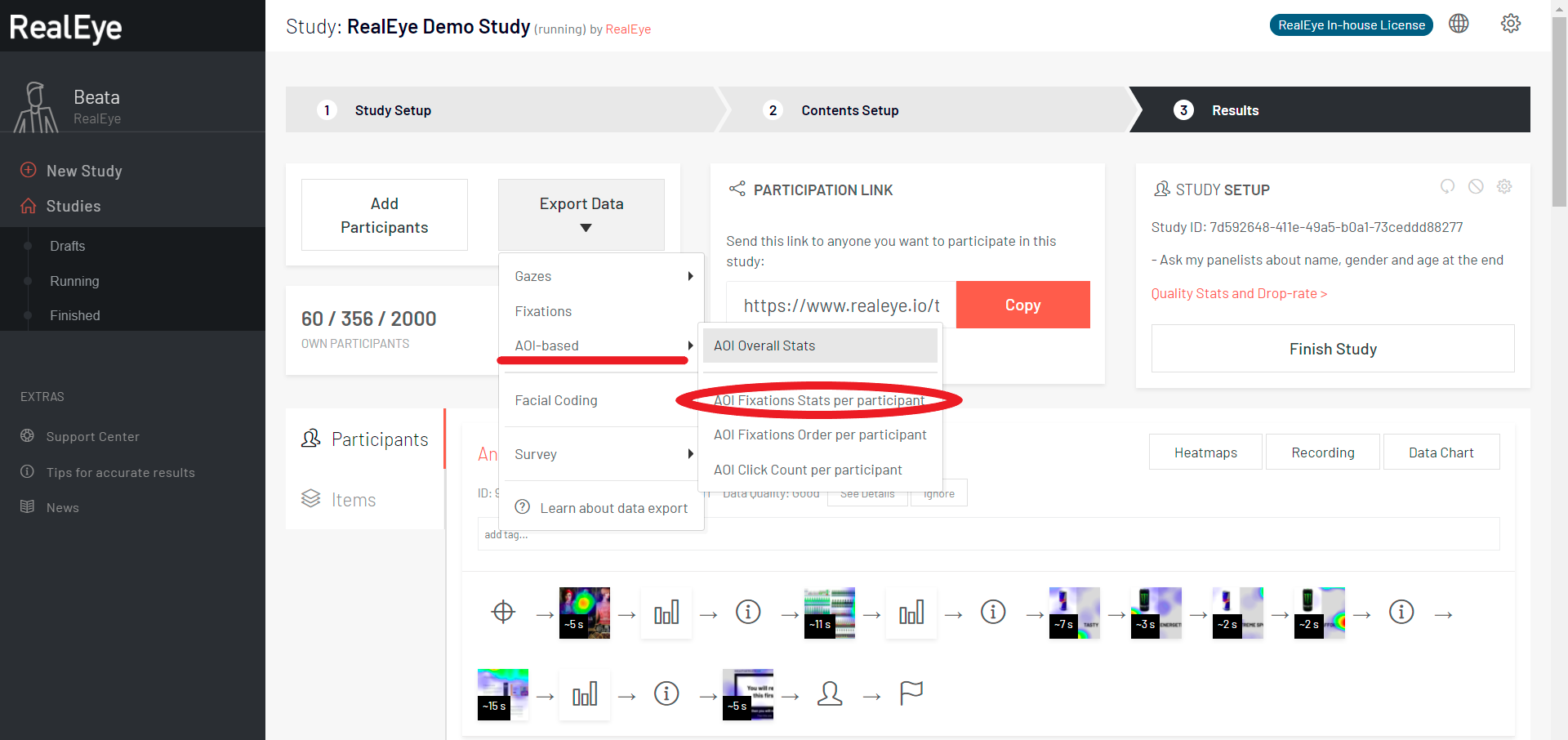# CSV file: AOI fixation stats

With RealEye you can not only download the Raw Data CSV, but also download the AOI data. You can obtain the CSV file from the main study dashboard or from the item heatmap dashboard - in this case you'll get data only for this particular item.The CSV contains the following fields:

• study_id - UUID,
• item_id - UUID,
• item_filename - the name of your file, as it was named while adding to study,
• tester_id - UUID,
• tester_external_id - (UUID) in case if you provide one by connecting the study with an external tool, i.e. a survey,
• tester_display_name - a name that a tester gives while asked about a name, gender, and age at the end of the study (if you have chosen that option), i.e. "Anna, Female, 27",
• 1 = very low,
• 2 = low,
• 3 = average,
• 4 = good,
• 5 = very good,
• 6 = perfect;
• 1 = very low,
• 2 = low,
• 3 = average,
• 4 = good,
• 5 = very good,
• 6 = perfect;
• test_item_display_order - a number indicating the order in which the items were displayed.
• aoi_id - UUID,
• aoi_name - the name that you gave the AOI while saving it,
• aoi_size_percents - AOI size expressed as the percentage value relative to the size of the entire image,
• aoi_time_range_from_ms - the start time from which the values of the AOI were calculated (you can set this time with the orange slider under the heatmap),
• aoi_time_range_to_ms - the end time to which the values of the AOI were calculated (you can set this time with the orange slider under the heatmap),
• aoi_fixation_total_count - number of fixations within this area,
• aoi_fixation average_duration_ms - the time that the average fixation lasted for (in milliseconds),
• aoi_fixation_ttff_ms - the amount of time that it takes participants (on average) to look at this area (in milliseconds),
• aoi_fixation_average_total_time_spent_ms - the amount of time that participants spent looking at this area (in milliseconds),
• aoi_fixation_first_fixation_average_duration_ms
• notes - this column contains:
• RealEye version,
• fixation filter settings:
• gazepoint interpolation (Hz) - to improve calculations we interpolate the data to 60 Hz;
• gazepoint interpolation max gap (ms) - we interpolate data if the timestamp gap between two input values is not bigger than this value (50 ms);
• gaze velocity threshold;
• min. fixation duration (Ms);
• noise reduction level.

See the CSV file for the sample study: